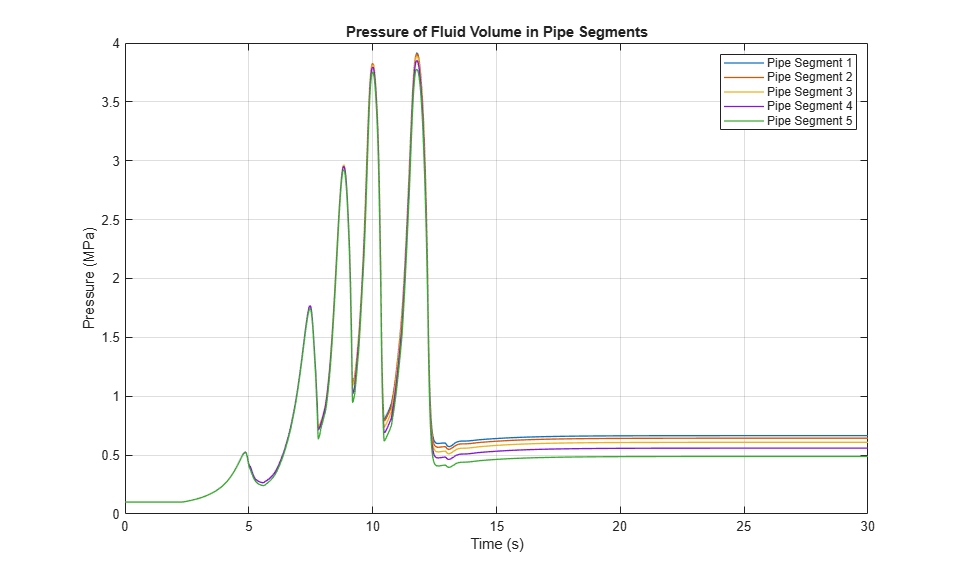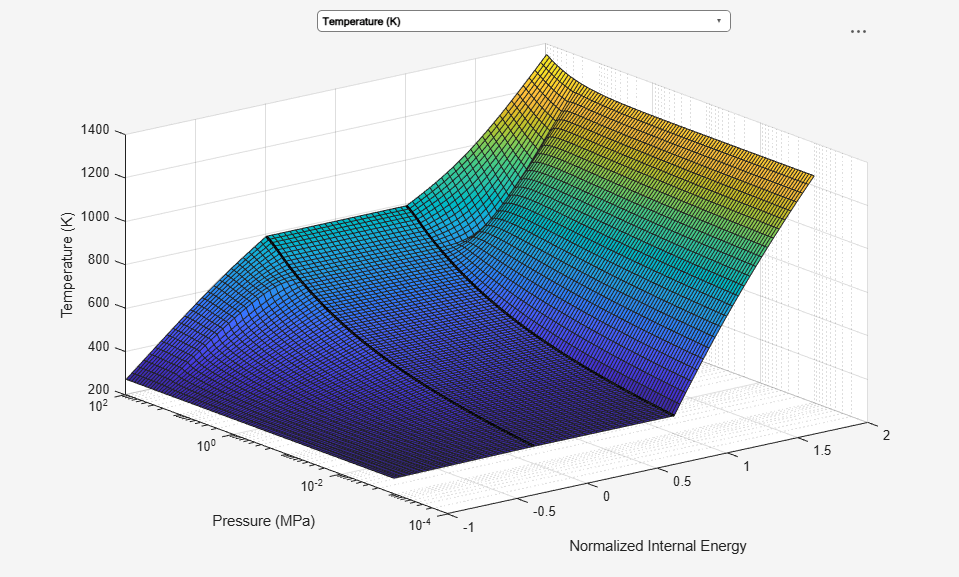# Fluid Vaporization in Pipe

This example shows how to model the vaporization of water to generate steam. Liquid water enters the pipe at 370 K at a rate of 1 kg/s. The pipe is heated to 1000 K, causing the water flowing inside pipe to saturate.

When liquid in a large fluid volume saturates, the vaporization process can produce a surge in fluid pressure. Breaking a pipe into multiple segments allows a smaller fluid volume in each segment to saturate one at a time, reducing the strength of the pressure spike.

### Model### Pipe Segment 1 Subsystem### Simulation Results from Scopes### Simulation Results from Simscape Logging

This plot shows the pressure of the fluid volume in each pipe segment. The five pressure spikes correspond to the saturation of the fluid volume in each pipe segment, starting with the last segment and progressing upstream to the first segment. When a liquid crosses the saturation boundary, its specific volume increases rapidly. If the fluid cannot evacuate the volume quickly enough, then pressure builds up inside the volume. In the Simscape™ Two-Phase Fluid Library, components such as Pipe (2P) represent the fluid as a lumped parameter model. This means that the entire fluid volume inside the component saturates at once, resulting in the pressure spikes seen in the plot.

In some models, these pressure spikes can produce unexpected behavior such as a rapid surge of reversed flow upstream. One way to mitigate the pressure spikes is to break a single long pipe into multiple shorter pipe segments. This allows the smaller fluid volume in each pipe segment to saturate one a time instead of all at once. Another way to mitigate the pressure spikes is to increase the value of the Phase change time constant parameter. In this example, the 10 m pipe is broken into five 2 m pipe segments in order to simulate water vaporizing into steam.This plot shows the specific enthalpy of the fluid volume in each pipe segment. The specific enthalpy is not available directly from the logged simulation data. However, it can be computed from the formula: h = u + p*v where u is the specific internal energy, p is the pressure, and v is the specific volume.### Fluid Properties

The following two figures plot the fluid properties of water as a function of pressure (p) and specific internal energy (u) and as a function of pressure (p) and normalized internal energy (unorm), respectively. The fluid is a

• subcooled liquid when -1 <= unorm < 0;

• two-phase mixture when 0 <= unorm <= 1;

• superheated vapor when 1 < unorm <= 2.

The fluid property data is provided as a rectangular grid in p and unorm. Therefore, the grid in terms of p and u is non-rectangular.

The water fluid property data can be found in `waterPropertyTables.mat`.